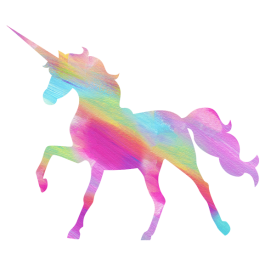# Factor Questions and Answers (Q&A)#### xiuliette

Highest common factor

Example HCF of 28 ad 91 is 7 because 7 times 13 is 91 and 7 times four is 28#### ruthcallcott

2x^2-18x

all the above answers are incorrect#### ruthcallcott

2x(x-9)

= (2xx)-(2x9)

= 2x square-18x

Therefore the Answer is: 2x square-18x#### Jeflin Lee

my name is Jeflin lee

YES! It is a factor of 2856.